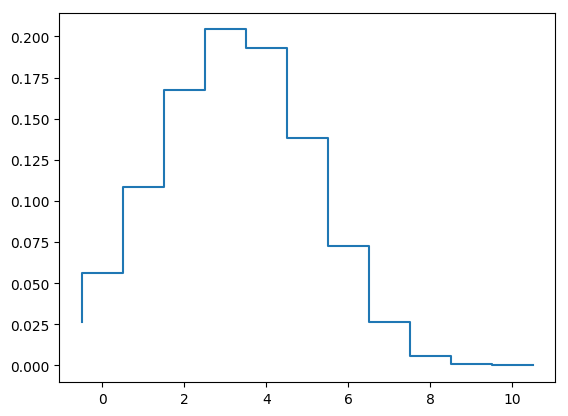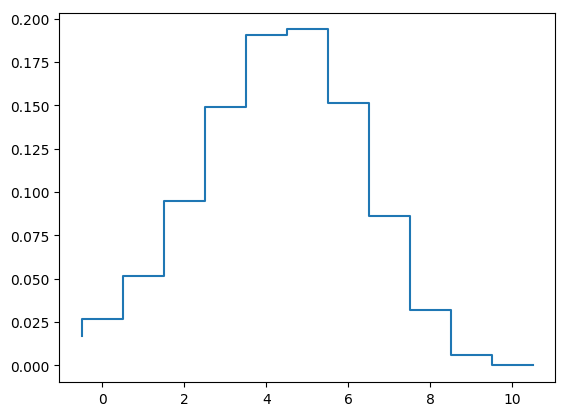# Disease dynamics in a population of households in Julia

Author: Tim Kinyanjui @timothykinyanjui

Date: 2018-10-04

# Set the required model parameters for the SIRS model with two levels of transmission - Within and between households
N = 10; # Household size - Change to 10 for final analysis
betaHH = 6; # Within household transmission parameter
betaG = 1; # Population wide transmission
gamma = 1; # Rate of recovery from infection
tau = 1; # Rate of loss of protection
params = [betaHH,gamma,tau,betaG,N]; # Put all the parameters together
time = (0.0, 30.0) # Simulation time - note it defined as a float
dim = dim = 0.5*(N+1)*(N+2); # Number of possible configurations - works for three epidemiological classes
y0 = vec(zeros(1,dim)); # Initial condition vector
y0[end-1] = 0.00000001;
y0[end] = 0.99999999;

function hhTransitions(N,dim)
# Function to generate transition matrices for household model
# Input: N is the household size

# Initialize things
Qinf = zeros(dim,dim);
Qrec = zeros(dim,dim);
Qext = zeros(dim,dim);
Qwane = zeros(dim,dim);
dataI = Array{Int64}(zeros(dim,3))
m = 0;
I = Array{Int64}(zeros(N+1,N+1))

# To help remember where to store the variables
for ss = 0:N
for ii = 0:(N-ss)
m = m + 1;
I[ss+1,ii+1] = m
end
end

# Describe the epidemiological transitions

# Counter for susceptibles
for ss = 0:N
# Counter for infecteds
for ii = 0:(N-ss)
# If susceptibles and infecteds are more than 1, then infection within the household can occur
if (ss > 0 && ii > 0)
Qinf[I[ss+1,ii+1],I[ss,ii+2]] = ii*ss/(N-1);
end

# If infecteds are more than 1, recovery can occur
if ii > 0
# Rate of recovery
Qrec[I[ss+1,ii+1],I[ss+1,ii]] = ii;
end

# For external infection - just keep track of susceptibles
if ss > 0
# Rate of within household infection
Qext[I[ss+1,ii+1],I[ss,ii+2]] = ss;
end

# Loss of protection hence becoming susceptible again. Possible if N-ss-ii = rr > 0
if (N-ss-ii) > 0
# Rate of loss of protection
Qwane[I[ss+1,ii+1],I[ss+2,ii+1]] = N-ss-ii;
end

# Store the relevant indices to help identify the household configurations
dataI[I[ss+1,ii+1],:] = [ss, ii, N-ss-ii];
end
end

Qinf = Qinf - diagm(vec(sum(Qinf,2)),0);
Qrec = Qrec - diagm(vec(sum(Qrec,2)),0);
Qext = Qext - diagm(vec(sum(Qext,2)),0);
Qwane = Qwane - diagm(vec(sum(Qwane,2)),0);

# Return
return Qinf, Qrec, Qext, Qwane, dataI
end
Qinf, Qrec, Qext, Qwane, dataI = hhTransitions(N,dim);

using DifferentialEquations
function rateSIRS(dy_dt,y0,params,time)

# Extract the parameters
betaHH = params;
gamma = params;
tau = params;
betaG = params;
N = params;

# Generate the transition matrices
Qinf, Qrec, Qext, Qwane, HHconfig = hhTransitions(N,dim);

# Combine within and external transitions
Q = betaHH*Qinf + gamma*Qrec + tau*Qwane + (betaG*((HHconfig[:,2]'*y0)/N)*Qext);

# Generate the rates of change for ODE solver
dy_dt .= (y0'*Q)';

# Alternatively this works too
#=for i=1:length(y0)
dy_dt[i] = y0'*Q[:,i]
end=#

end

# Define the ODE problem
prob = ODEProblem(rateSIRS,y0,time,params);

# Solve
sol = solve(prob);

using Plots

Iconfig = dataI[:,2];
infProp = zeros(length(sol.t),1);

# Prepare the plots
for i = 1:length(sol.t)
infProp[i,1] = sol[:,i]'*Iconfig/N;
end

# Total infectious in the population
plot(sol.t,infProp,color="blue",xlabel="Time",ylabel="Proportion infectious",label=["Mean infection"],ylims=[0, 1])# Household profile at endemic prevalence
# Prepare the plots
using PyPlot
yprop = zeros(N+1,length(sol.t))
for j = 1:length(sol.t)
for i = 1:N+1
index = find(Iconfig.==i-1);
yprop[i,j] = sum(sol[index,j])
end
end
step(-0.5:1:10.5,[yprop[:,length(sol.t)];0])1-element Array{PyCall.PyObject,1}:
PyObject <matplotlib.lines.Line2D object at 0x7fe91ef1c210>

# Household profile at peak prevalence
# Prepare the plots
x = find(infProp.==maximum(infProp))
step(-0.5:1:10.5,[yprop[:,x];0]);